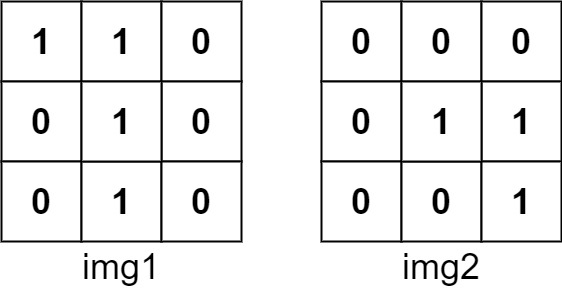# 835. Image Overlap

Medium
You are given two images, `img1` and `img2`, represented as binary, square matrices of size `n x n`. A binary matrix has only `0`s and `1`s as values.
We translate one image however we choose by sliding all the `1` bits left, right, up, and/or down any number of units. We then place it on top of the other image. We can then calculate the overlap by counting the number of positions that have a `1` in both images.
Note also that a translation does not include any kind of rotation. Any `1` bits that are translated outside of the matrix borders are erased.
Return the largest possible overlap.
Example 1:Input: img1 = [[1,1,0],[0,1,0],[0,1,0]], img2 = [[0,0,0],[0,1,1],[0,0,1]]
Output:
3
Explanation:
We translate img1 to right by 1 unit and down by 1 unit.
The number of positions that have a 1 in both images is 3 (shown in red).
Example 2:
Input: img1 = [], img2 = []
Output:
1
Example 3:
Input: img1 = [], img2 = []
Output:
0
Constraints:
• `n == img1.length == img1[i].length`
• `n == img2.length == img2[i].length`
• `1 <= n <= 30`
• `img1[i][j]` is either `0` or `1`.
• `img2[i][j]` is either `0` or `1`.

### 解題

Runtime: 84 ms, faster than 50.00%
Memory Usage: 6.1 MB, less than 50.00%

func largestOverlap(img1 [][]int, img2 [][]int) int {
length := len(img1)
arr1 := make([]int, 0)
arr2 := make([]int, 0)
for i:= 0; i<length; i++ {
for j:=0; j<length; j++ {
if img1[i][j]==1 { arr1 = append(arr1, i*100+j) }
if img2[i][j]==1 { arr2 = append(arr2, i*100+j) }
}
}
count := make(map[int]int)
ans := 0
for i:=0; i<len(arr1); i++ {
for j:=0; j<len(arr2); j++ {
count[arr1[i]-arr2[j]]++
if count[arr1[i]-arr2[j]] > ans {
ans = count[arr1[i]-arr2[j]]
}
}
}
return ans
}